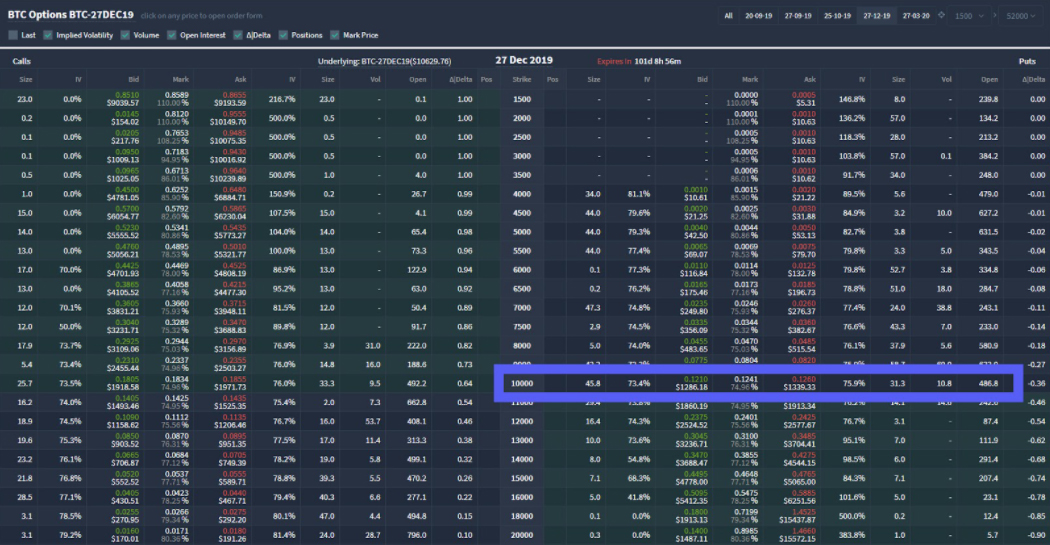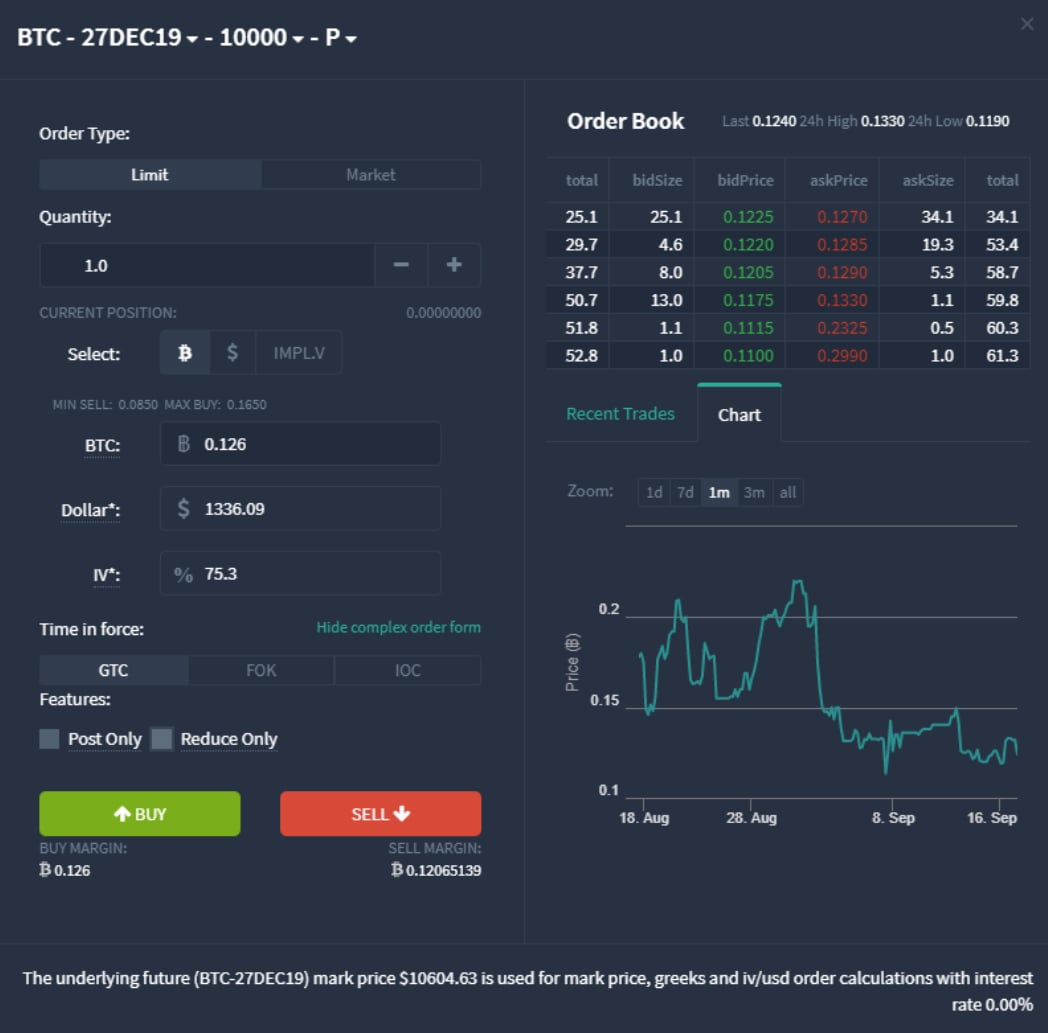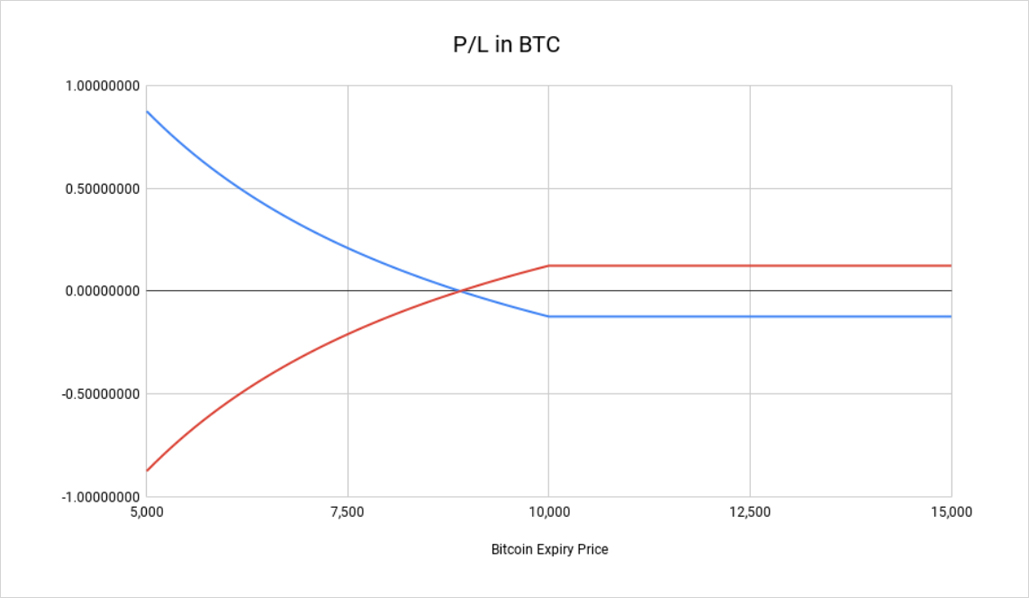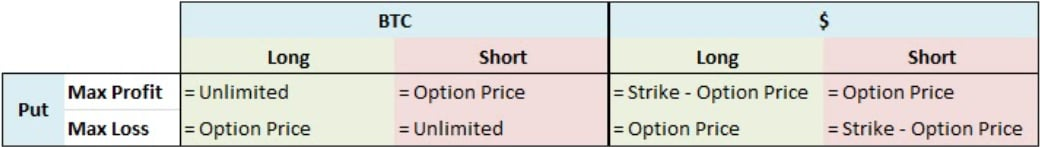In this lesson we will be focusing specifically on cryptocurrency put options. We’ll be looking at how they work, their payout structures, how to calculate their profit/loss and how to calculate their break even point.

Let’s have a brief recap of the 5 basic parameters that make up an option:

• Underlying asset – The underlying asset, the price of which is being speculated on, for example Bitcoin
• Option type – Call or put
• Expiry date – The date the option will expire and be exercised
• Strike price – The price at which the option buyer has the right to trade at on the expiry date
• Option price (aka option premium) – The price the buyer pays to the seller for the right to trade the asset at the strike price on the expiry date

A put option is the right to sell the underlying asset at the strike price on the expiry date.

All the options on Deribit are European style which means they are only exercised at expiry, however this does not stop traders being able to buy and sell the option before expiry.

They are also cash settled which means when they are exercised, it is only the profits that are paid. For example if a Bitcoin put option with a strike price of \$10,000 expires with the Bitcoin price at \$8,000, then \$2,000 would be paid from the seller to the buyer. On Deribit this \$2,000 is paid in Bitcoin so this would be 0.25 BTC (\$2000/\$8,000).

## Profit/loss for a Bitcoin put option

For a put option, if the price expires above the strike price, then the option expires out of the money and therefore worthless. The buyer’s only loss is the premium paid, and of course the buyer’s loss is the seller’s profit.

However, if the price expires below the strike price, this option is in the money and has some value. The seller is then required to pay to the buyer the difference between the expiry price and the strike price. As all balances on Deribit are cryptocurrency, the difference in dollars will of course be converted to BTC.

The buyer’s profit/loss in BTC is calculated as:
= ((Strike Price – BTC Price)/BTC Price) – Option Price

The seller’s profit/loss of course is the opposite, and can be calculated as:
= Option Price – ((Strike Price – BTC Price)/BTC Price)

### Example

Taking a look at the Bitcoin option chain for the December 2019 expiry, we can see here the \$10,000 put option.Let’s work through using this option as an example to calculate and visualise the profit/loss and breakeven point. We will assume a quantity of 1 and use a price of 0.126 BTC as displayed here.So the buyer has paid 0.126 BTC to the seller for this option and held the option to expiry.

Scenario 1
At 0800 UTC on 27DEC19 the option expires and the settlement price is \$12,000.

The settlement price of \$12,000 is above the strike price of \$10,000 so the option has expired out of the money and therefore worthless.

The buyer paid 0.126 BTC for this option and received nothing in return, so they have made a loss of 0.126 BTC.

The seller collected 0.126 BTC for the option and has not had to pay anything out, so they have made a profit of 0.126 BTC

Scenario 2
At 0800 UTC on 27DEC19 the option expires and the settlement price is \$8,000.

The settlement price of \$8,000 is below the strike price of \$10,000 so the option has a value of \$2,000 (10,000 – 8,000). This must be paid by the seller to the buyer.

The buyer’s total profit/loss can be calculated as follows:
= ((Strike Price – BTC Price) / BTC Price) – Option Price
= ((10000 – 8000) / 8000) – 0.126
= 0.25 – 0.126
= 0.124 BTC

The seller’s loss will of course just be the negative of this, but can be calculated as follows:
= Option Price – ((Strike Price – BTC Price) / BTC Price)
= 0.126 – ((10000-8000) / 8000)
= 0.126 – 0.25
= 0.124

More generally we can plot the profit/loss of an option for both buyer and seller on a chart like so:This chart shows the \$10,000 put option we selected and used in the examples, with the expiry price along the x-axis, and the profit/loss in BTC on the y-axis. The buyer’s profit/loss is displayed in blue and the seller’s profit/loss is displayed in red.

As you would expect the inflection point is the strike price at \$10,000. Above this point the profit/loss is fixed to the premium paid of 0.126 BTC. This is one of the most appealing features of being long a put option. No matter how high the price rises, the buyer can never lose more than they paid for the option. They still have short exposure similar to being short a futures contract for example, but with a fixed risk. It’s not all good news for the buyer though.

## Breakeven point of cryptocurrency put options

Notice where the profit/loss lines cross the x-axis. This is the breakeven point. Notice how even though the put option has a strike price of \$10,000, the breakeven point is considerably lower than this. This means the price needs to move quite far in the right direction before the position is profitable at expiry. The higher the price paid for the option, the further away from the strike price the breakeven price will be.

The put option is at breakeven when:
((Strike Price – BTC Price) / BTC Price) – Option Price = 0

So rearranging this formula to solve for BTC Price gives us:
Put Breakeven BTC Price = Strike Price / (1 + Option Price)

Breakeven Example 1
Taking the previous example where the option price was 0.126 BTC and the strike price was \$10,000, we can calculate the breakeven price precisely as follows:

Breakeven Price
= 10000 / (1 + 0.126)
= 10000 / 1.126
= \$8,880.99 (rounded to the nearest penny)

Breakeven Example 2
Taken from the same screenshot as the first example, let’s calculate the breakeven price of the \$7,000 put assuming a price of 0.026 BTC.

Breakeven Price
= 7000 / (1 + 0.026)
= 7000/ 1.026
= \$6,822.61 (rounded to the nearest penny)

## Maximum profit/loss

The put buyer’s maximum loss is always limited to the option price paid. This also means the put seller’s maximum profit is also limited to the option price collected.

Maximum profit for the buyer when measured in BTC is unlimited. As the underlying Bitcoin price continues to fall, the amount of BTC required to pay the USD amount owed increases dramatically. This is bad news for the seller as it means their maximum loss measured in BTC is unlimited when selling Bitcoin put options.

Here you can see a comparison of the maximum profit/loss for the put option buyer (long) and put option seller (short), measured in both BTC and USD.## Pros and cons of put options

A trader may wish to buy a put option instead of shorting a futures contract if they believe the price is going to go down, but they wish to have a fixed risk. It is possible of course to use a stop loss when short a futures contract to limit your risk, however a put option has one very big advantage in comparison.

The put option can not be stopped out or liquidated. If the price spikes up even temporarily the trader who is short the futures contract may be stopped out, unable to benefit from subsequent price decreases. The trader who is long the put option though will still be in the trade, and so still able to benefit it the price then falls.

Stop losses also have the added risk of slippage, where due to the violent nature of the price movement and thin orderbooks the stop loss order executes at a worse price then desired, leading to a larger than expected loss. Long options positions do not have this problem as the maximum loss is limited to the premium paid and can never go higher.

Of course there is also a downside to buying put options. Firstly, a premium must be paid. As shown earlier this means the breakeven price is lower than the strike price, sometimes considerably so. How far away this is depends on the amount paid for the option.

Secondly, the option has a time limit. The expiry date of the option means the clock is ticking as soon as the option is purchased. If the underlying price fails to move sufficiently by the expiry date, the trade is over and will finish as a loser. So the put option buyer must not only be correct about the direction, but also the timing.

This brings us nicely onto why a trader would choose to sell a put option. The put option seller benefits not only when the underlying price rises, but also when it fails to fall fast enough before the expiry date. If the price expires above the strike price, the seller gets to keep the entire premium. Even if the price falls though, they can still make some profit as long as it doesn’t fall past the breakeven point.

The downside for the seller being that they do not get the benefit of having a fixed risk. If the underlying price keeps falling well past the strike price, their losses will also continue to rise. With put options that use the asset itself as collateral (for example using Bitcoin as collateral for Bitcoin options) this can quickly become considerably more than the seller intended to risk when measured in Bitcoin.

## Summary

• A put option gives the buyer a way to have short exposure to the price of an asset with a fixed risk.
• The cost for this fixed risk position is the premium the buyer pays to the seller.
• The buyer must also be correct about the timing of the fall in price.
• The breakeven price for a put option will always be below the strike price. How far below depends on the price paid for the option.

AUTHOR(S)CryptarbitrageMrJozza